Verifying properties of a determinant

Chapter 4 Class 12 Determinants
Concept wise

There are some properties of Determinants, which are commonly used

## Property 1

The value of the determinant remains unchanged if it’s rows and

columns are interchanged

(i.e. |𝐴 𝑇 | = |A|)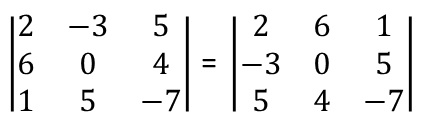Check Example 6

## Property 2

If any two rows (or columns) of a determinant are interchanged then sign of determinant changes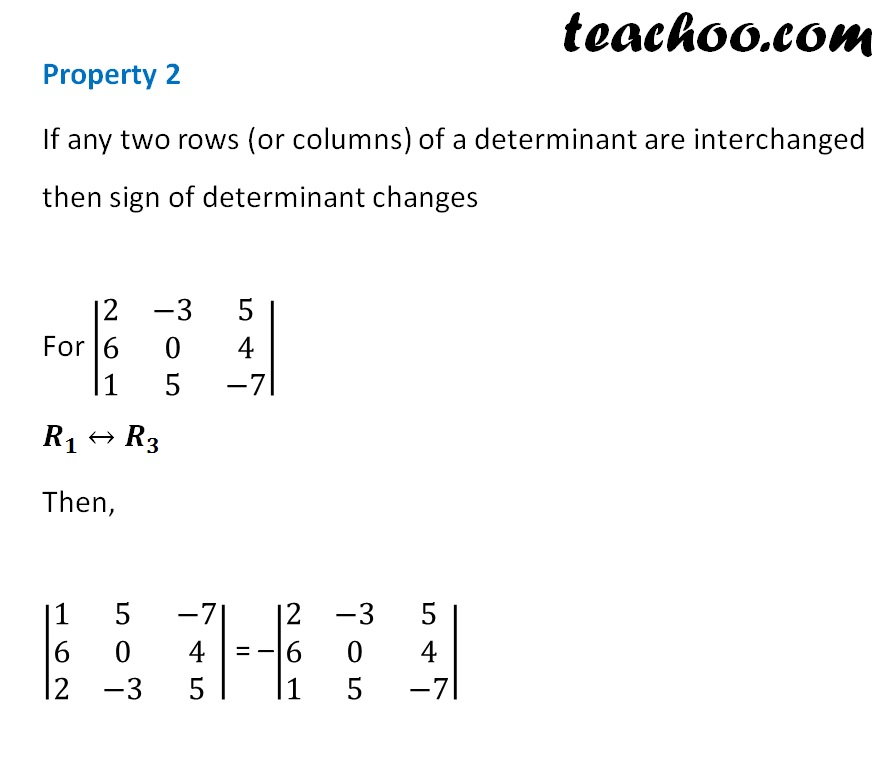Check Example 7

## Property 3

If all elements of a row (or column) are zero, determinant is 0.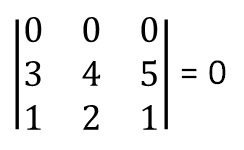## Property 4

If any two rows (or columns) of a determinant are identical, the value of determinant is zero.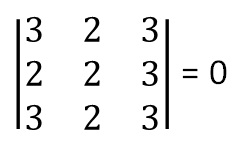Check Example 8 for proof

## Property 5

If each element of a row (or a column) of a determinant is multiplied by a constant k, then determinant’s value gets multiplied by k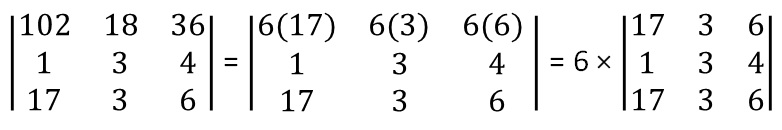Check Example 9

## Property 6

If elements of a row or column of a determinant are expressed as

sum of two (or more) terms,

then the determinant can be expressed as sum of two (or more) determinants.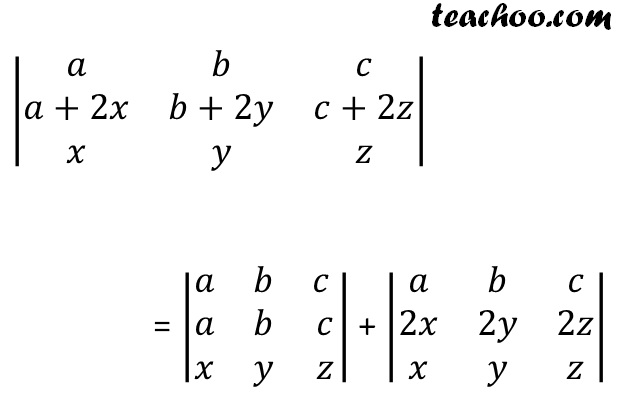Check Example 10 for proof

## Property 7

If in a determinant all the elements above or below the diagonal is

zero,

then value of the determinant is equal to product of the diagonal elements.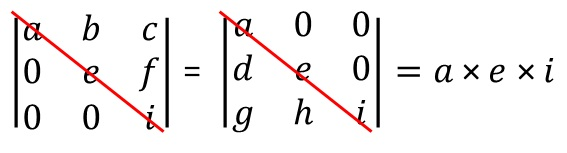Learn in your speed, with individual attention - Teachoo Maths 1-on-1 Class